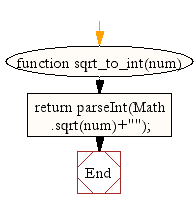# JavaScript: Cast a square root of a number to an integer

## JavaScript Math: Exercise-30 with Solution

Write a JavaScript function to cast the square root of a number to an integer.

Test Data:
console.log(sqrt_to_int(17));
4

Sample Solution:-

HTML Code:

``````<!DOCTYPE html>
<html>
<meta charset="utf-8">
<title>JavaScript function to cast a square root of a number to an integer.</title>
<body>

</body>
</html>
```
```

JavaScript Code:

``````function sqrt_to_int(num)
{
return parseInt(Math.sqrt(num)+"");
}

console.log(sqrt_to_int(17));
```
```

Sample Output:

```4
```

Flowchart:Live Demo:

See the Pen javascript-math-exercise-30 by w3resource (@w3resource) on CodePen.